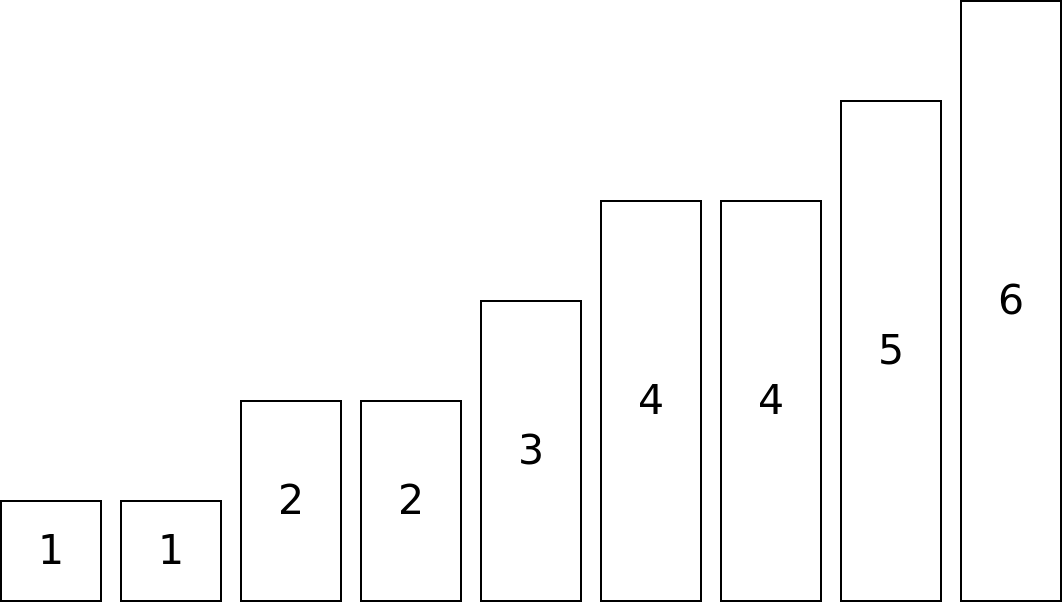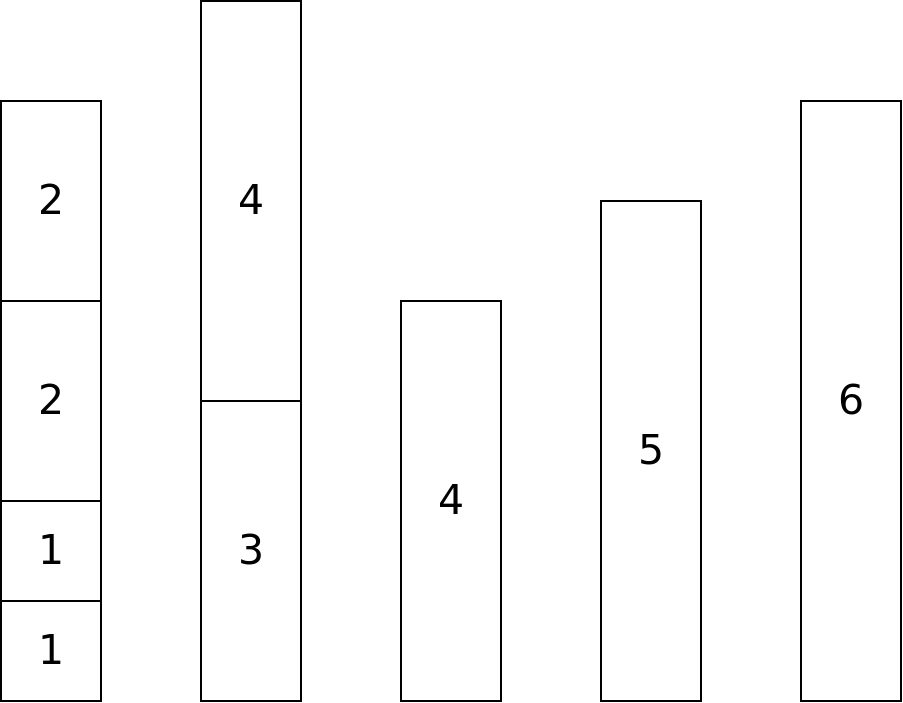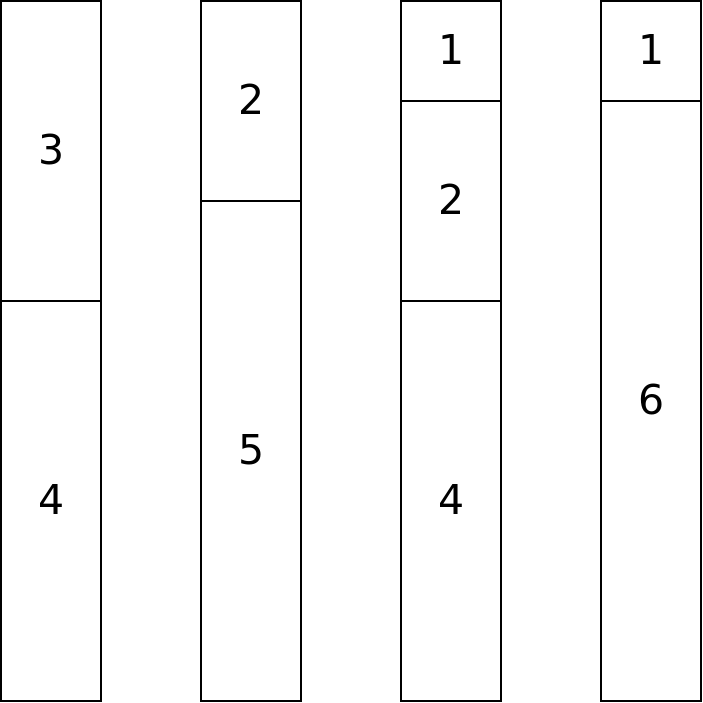# Bin Packing Approximation

## Objective

To gain experience writing parallel programs, and learn about genetic approximation algorithms.

## The Bin Packing Problem

The bin packing problem is a well-known optimization problem. In this problem, you have $N$ items of different sizes. You want to pack the items into bins, using the fewest bins possible.

For instance, imagine we have the following items:We want to pack these items into bins such that we use as few bins as possible. Let's say the bin size is 7. That means that the total size of the items in any one bin must be 7 or less.

A simple greedy solution to the problem is to just take the items, one by one, and put them into a bin they will fit in. If they don't fit in any, we start a new bin. If we follow this algorithm for the items above, we will use 5 bins:However in this case, there is a better solution using only 4 bins:Bin packing is a very hard, $NP$-Complete problem. One way of viewing the solution to the problem is to find the ordering of the $N$ items, in which the greedy solution produces the correct result. For example, if we use that method in the items in the order:

4, 3, 5, 2, 4, 2, 1, 6, 1

Then we will arrive at the optimal solution. The problem is that with $N$ items, there are $N!$ many orderings. The brute force solution, then, is to try to pack the items in all $N!$ orders, and see which one was best. This is not feasible for large values of $N$. For example, when $N = 100$, $N! = 9.33 \times 10^{157}$. For comparison, the number of atoms in the observable universe is estimated to be about $10^{80}$.

Rather than find an exact solution to bin packing, what most do is find an approximate solution. An approximation algorithm is one which finds a "good enough" solution to a problem which may or may not be the best one possible.

## Genetic Algorithms

There is a type of approximation algorithm that can be applied to a wide number of optimization problems called genetic algorithms. A genetic algorithm borrows concepts from biological evolution to iteratively improve upon a set of solutions.

In a genetic algorithm, we maintain a population of solutions. In each step of the algorithm, we determine the fitness of each member of the population - how likely it is to survive. Then the members of the population who are least fit are eliminated. Then new members are created by modifying the most fit members of the population.

If we use a genetic algorithm to approximate bin packing, the population could consist of orderings of the items. The fitness will be the number of bins used to store those items using the greedy algorithm. The most fit members of the population which use the fewest bins.

When the algorithm starts, the population consists of entirely random sequences. Sequences which use more bins will be removed. The new members of the population can be created by randomly mutating the sequences with the fewest bins: randomly changing the order of some of the items. Sometimes, entirely new random sequences can be created which represent new species - this can avoid the process being stuck in local minimums.

The exact details are left up to you:

1. How many sequences are in the population?
2. What percentage die at each iteration?
3. What percentage of the best solutions are new members based on?
4. How exactly are new members mutated?
5. Are entirely new random sequences introduced? And how often?
6. When does this process stop?

When the genetic algorithm finishes, the most fit member of the population is given as the approximate solution.

## Parallel Genetic Algorithms

Genetic algorithms are good candidates for being parallelized. There are multiple approaches to this:

1. Each process acts as an isolated continent: the solutions evolve independently and the best solution from any process is taken at the end.
2. Each process acts as a region: the solutions evolve there, but there is migration where members move from one process to another.
3. Each process represents one individual in the population: the process calculates the fitness of the individual and is responsible for mutating it when needed given information about the fit individual it should be based on.

Which approach you take is up to you.

## Program Details

Your job is to use a parallel genetic algorithm, using either Pthreads, OpenMP, MPI or CUDA, to solve instances of the bin packing problem, using some variant of the parallel genetic algorithm described above.

Your program should read in the input file as the first and only input to the program.

The first line of the input file gives the size of each bin. For each test case, there is only 1 size of bin to pack, but each test case has a different size bin.

The second line gives the number of items, $N$. Following that are $N$ lines, each giving the size of one item to be packed.

There are three test cases that you can work with:

Test CaseItemsOptimal Bins
micro.txt83
small.txt297
large.txt150???

The first test case has only 8 items, which have $8! = 40320$ different orderings. The optimal packing is tight - each bin is totally filled to capacity. The second test case has 29 items which can fit into 7 bins. There are many more possible packings of these items, but there is some extra space in the packing. Your program should be able to find the optimal solution for these two test cases.

The third test case has 150 items, which have a colossal number of possible packings. I don't know the optimal number of bins to pack these numbers. Finding an exact solution to this instance of the problem would take an inordinate amount of time on our computer systems. Instead, we'll try to find the best solution we can using the genetic algorithm. Note that the number of bins can't be less than 52, due to the total weight of the items - but the actual minimum is likely a bit higher than that.

If you are using CUDA, you can use the cuRAND library to find random numbers. This page details how the cuRAND library works.

Your program should output the best packing you found along with the total number of bins. The output should include which items go in which bins, the size of each bin, along with the total bins used. Below is an example (for the micro test case):

\$ ./pack micro.txt
Bin 1: 5, 9, 11 (sum = 25)
Bin 2: 15, 10 (sum = 25)
Bin 3: 6, 11, 8 (sum = 25)
Total weight is 75.
Packed into 3 bins.


The student who finds the best approximation for the largest test case will have a 25% bonus added to their grade for this assignment (e.g. they could get a 125%). The student with the second best approximation will have a 10% bonus.

## Tips

Below are some ideas for how to go about writing the program, which you do not have to follow:

• Start with a sequential solution, and parallelize it once it's working.
• Start by reading the input file in, storing the items in an array of ints.
• Next, write a function to randomly shuffle the array (a simple technique is to swap two random items a bunch of times).
• Create your "population" by randomly shuffling the items, and copying them into an array of arrays.
• Next, write a function to apply the greedy algorithm - looping through an array of items and seeing how many bins they take in that order.
• Then, write a function to loop through all the solutions in the population and choose the best one.
• At this point, you should be able to get some kind of answer to the packing problem.
• In order to "evolve" towards better answers, write code to loop some number of times. Inside this loop, apply the genetic algorithm - find the worst solutions in the population and replace them, either with new random ones or with modified versions of the best ones.
• Finally, parallelize the program. The simplest way is to just run the whole process in parallel and find the best overall answer at the end. As discussed, you could do fancier things as well.

## Submitting

In addition to your code, you should include a brief description of your program. This can be a separate document or just the message of an email. The description should include:

• How your parallel genetic algorithm works including the population size, the way that the population is parallelized, how many unfit members are killed, how you create new members etc.